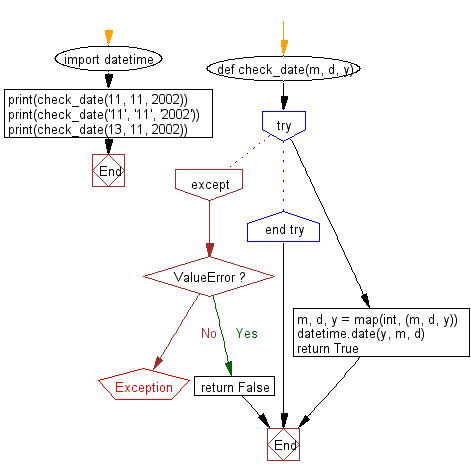﻿ Python: Validate a Gregorian date - w3resource# Python: Validate a Gregorian date

## Python Datetime: Exercise-53 with Solution

Write a Python program to validate a Gregorian date. The month is between 1 and 12 inclusive, the day is within the allowed number of days for the given month. Leap year’s are taken into consideration. The year is between 1 and 32767 inclusive.

Sample Solution:

Python Code:

``````import datetime
def check_date(m, d, y):
try:
m, d, y = map(int, (m, d, y))
datetime.date(y, m, d)
return True
except ValueError:
return False

print(check_date(11, 11, 2002))
print(check_date('11', '11', '2002'))
print(check_date(13, 11, 2002))
```
```

Sample Output:

```True
True
False
```

Flowchart:## Visualize Python code execution:

The following tool visualize what the computer is doing step-by-step as it executes the said program:

Python Code Editor:

What is the difficulty level of this exercise?

Test your Python skills with w3resource's quiz

﻿

## Python: Tips of the Day

Generator comprehension:

```# A generator comprehension is the lazy version of a list comprehension.
>>> m = (x ** 2 for x in range(5))
>>> m
<generator object <genexpr> at 0x108efe408>
>>> list(m)
[0, 1, 4, 9, 16]

>>> m = (x ** 2 for x in range(5))
>>> next(m)
0
>>> list(m)
[1, 4, 9, 16]
```